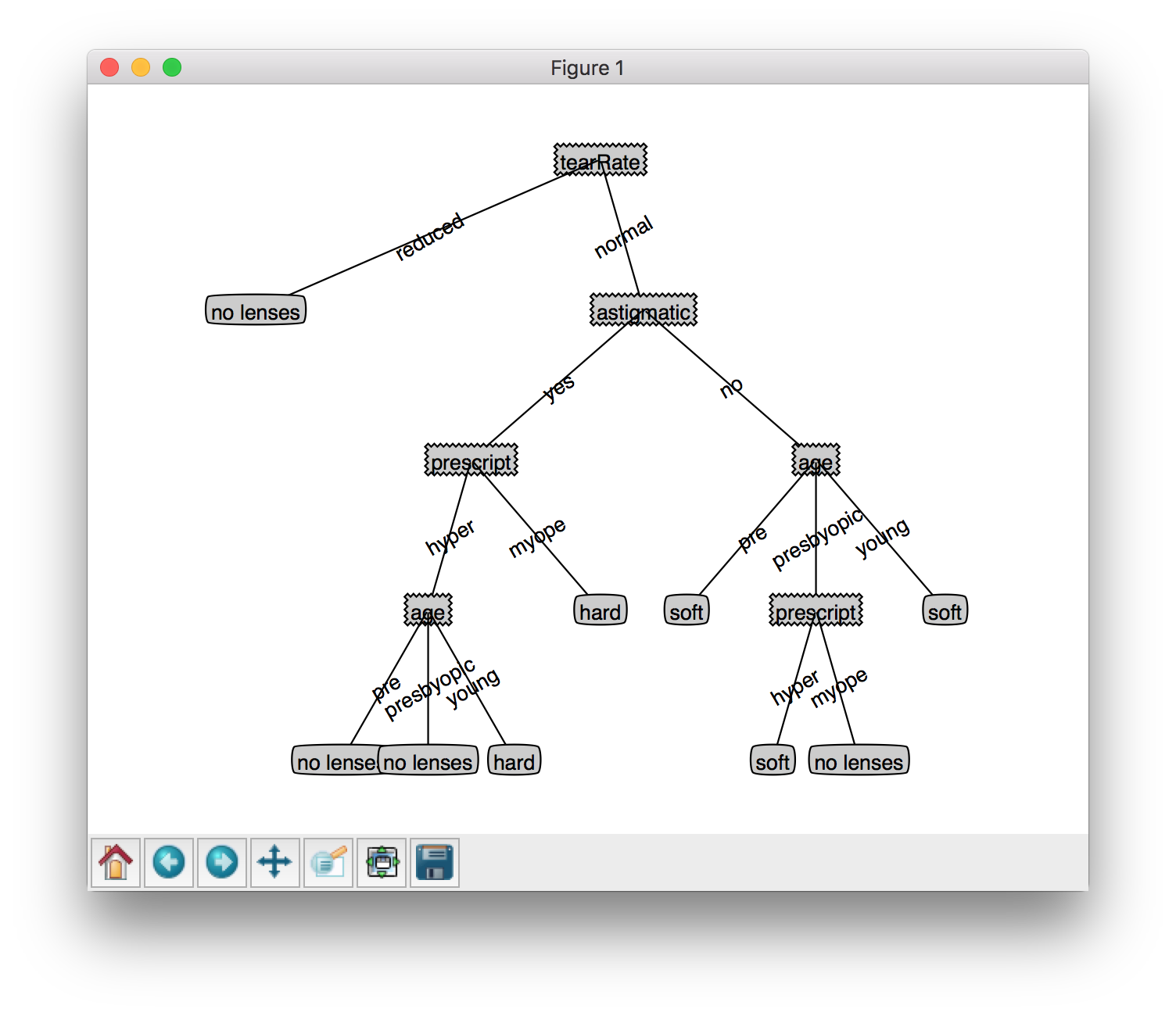# 机器学习实战之决策树

Posted by jjx on January 22, 2017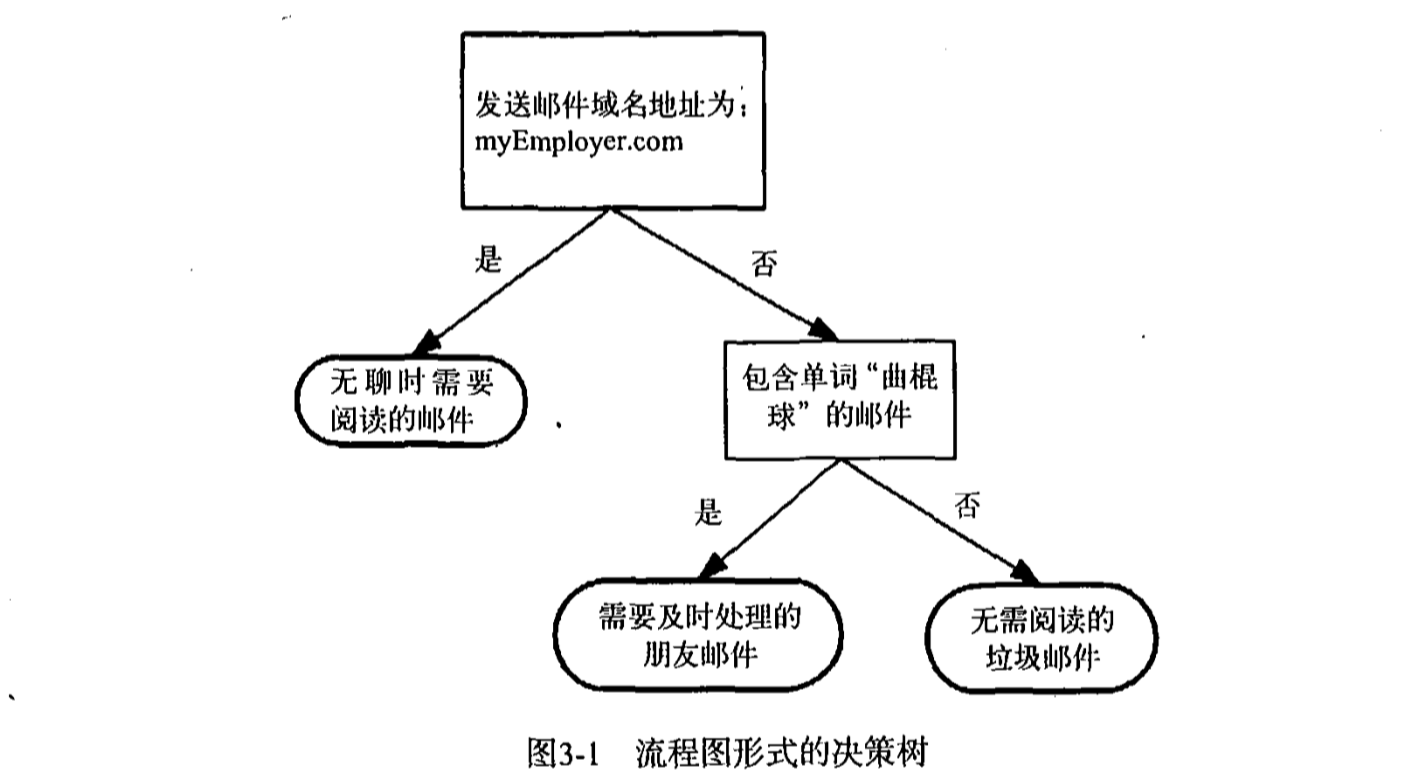#### 决策树的构造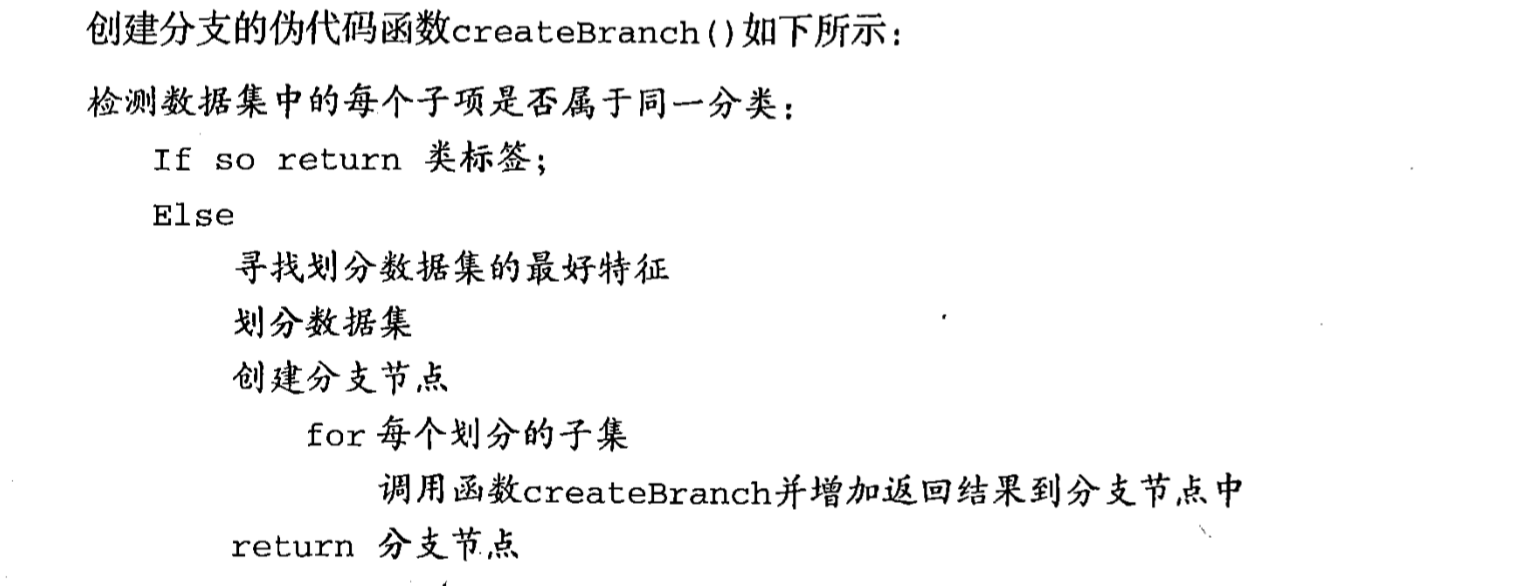(1)收集数据:可以使用任何方法。
(2)准备数据:树构造算法只适用于标称型数据,因此数值型数据必须离散化。
(3)分析数据:可以使用任何方法,构造树完成之后,我们应该检查图形是否符合预期。
(4)训练算法:构造树的数据结构。
(5)测试算法:使用经验树计算错误率。
(6)使用算法:此步骤可以适用于任何监督学习算法,而使用决策树可以更好地理解数据的内在含义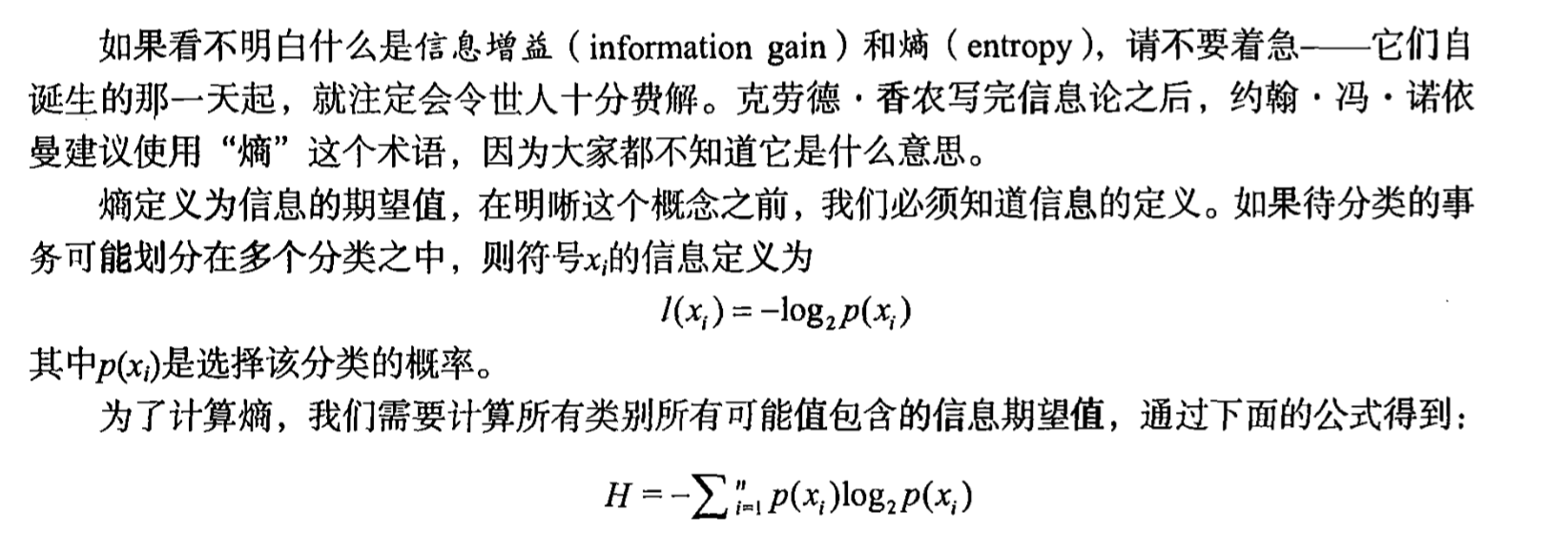def calcShannonEnt(dataSet):
numEntries = len(dataSet)
labelCounts = {}
for featVec in dataSet:  # the the number of unique elements and their occurance
currentLabel = featVec[-1]
if currentLabel not in labelCounts.keys(): labelCounts[currentLabel] = 0
labelCounts[currentLabel] += 1
shannonEnt = 0.0
for key in labelCounts:
prob = float(labelCounts[key]) / numEntries
shannonEnt -= prob * log(prob, 2)  # log base 2
return shannonEnt


def splitDataSet(dataSet, axis, value):
retDataSet = []
for featVec in dataSet:
if featVec[axis] == value:
reducedFeatVec = featVec[:axis]  # chop out axis used for splitting
reducedFeatVec.extend(featVec[axis + 1:])
retDataSet.append(reducedFeatVec)
return retDataSet


def chooseBestFeatureToSplit(dataSet):
numFeatures = len(dataSet) - 1  # the last column is used for the labels
baseEntropy = calcShannonEnt(dataSet)
bestInfoGain = 0.0;
bestFeature = -1
for i in range(numFeatures):  # iterate over all the features
featList = [example[i] for example in dataSet]  # create a list of all the examples of this feature
uniqueVals = set(featList)  # get a set of unique values
newEntropy = 0.0
for value in uniqueVals:
subDataSet = splitDataSet(dataSet, i, value)
prob = len(subDataSet) / float(len(dataSet))
newEntropy += prob * calcShannonEnt(subDataSet)
infoGain = baseEntropy - newEntropy  # calculate the info gain; ie reduction in entropy
if (infoGain > bestInfoGain):  # compare this to the best gain so far
bestInfoGain = infoGain  # if better than current best, set to best
bestFeature = i
return bestFeature  # returns an integer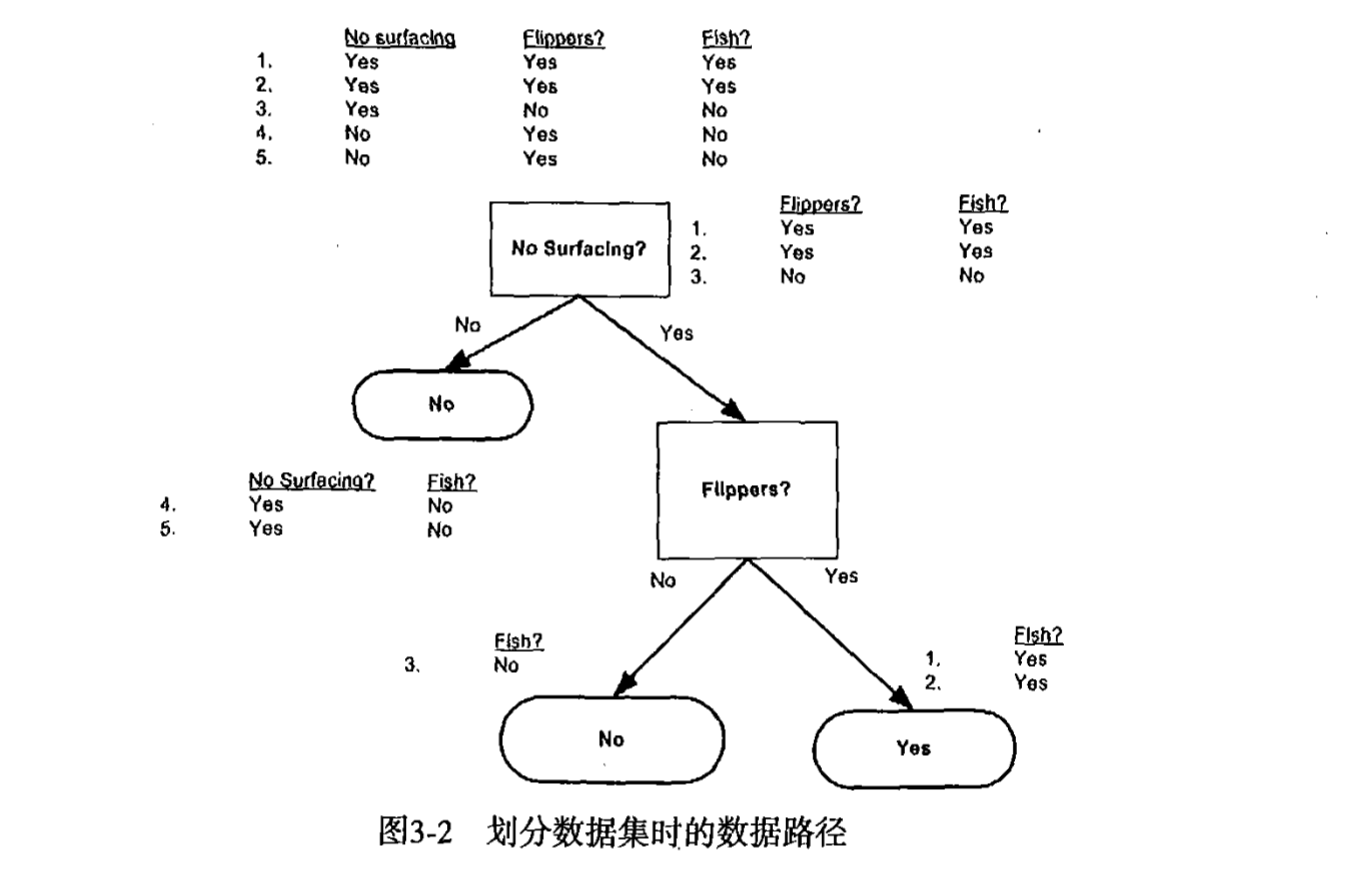def majorityCnt(classList):
classCount = {}
for vote in classList:
if vote not in classCount.keys(): classCount[vote] = 0
classCount[vote] += 1
sortedClassCount = sorted(classCount.iteritems(), key=operator.itemgetter(1), reverse=True)
return sortedClassCount


def createTree(dataSet, labels):
classList = [example[-1] for example in dataSet]
if classList.count(classList) == len(classList):
return classList  # stop splitting when all of the classes are equal
if len(dataSet) == 1:  # stop splitting when there are no more features in dataSet
return majorityCnt(classList)
bestFeat = chooseBestFeatureToSplit(dataSet)
bestFeatLabel = labels[bestFeat]
myTree = {bestFeatLabel: {}}
del (labels[bestFeat])
featValues = [example[bestFeat] for example in dataSet]
uniqueVals = set(featValues)
for value in uniqueVals:
subLabels = labels[:]  # copy all of labels, so trees don't mess up existing labels
myTree[bestFeatLabel][value] = createTree(splitDataSet(dataSet, bestFeat, value), subLabels)
return myTree


#### python中使用matplotlib绘制树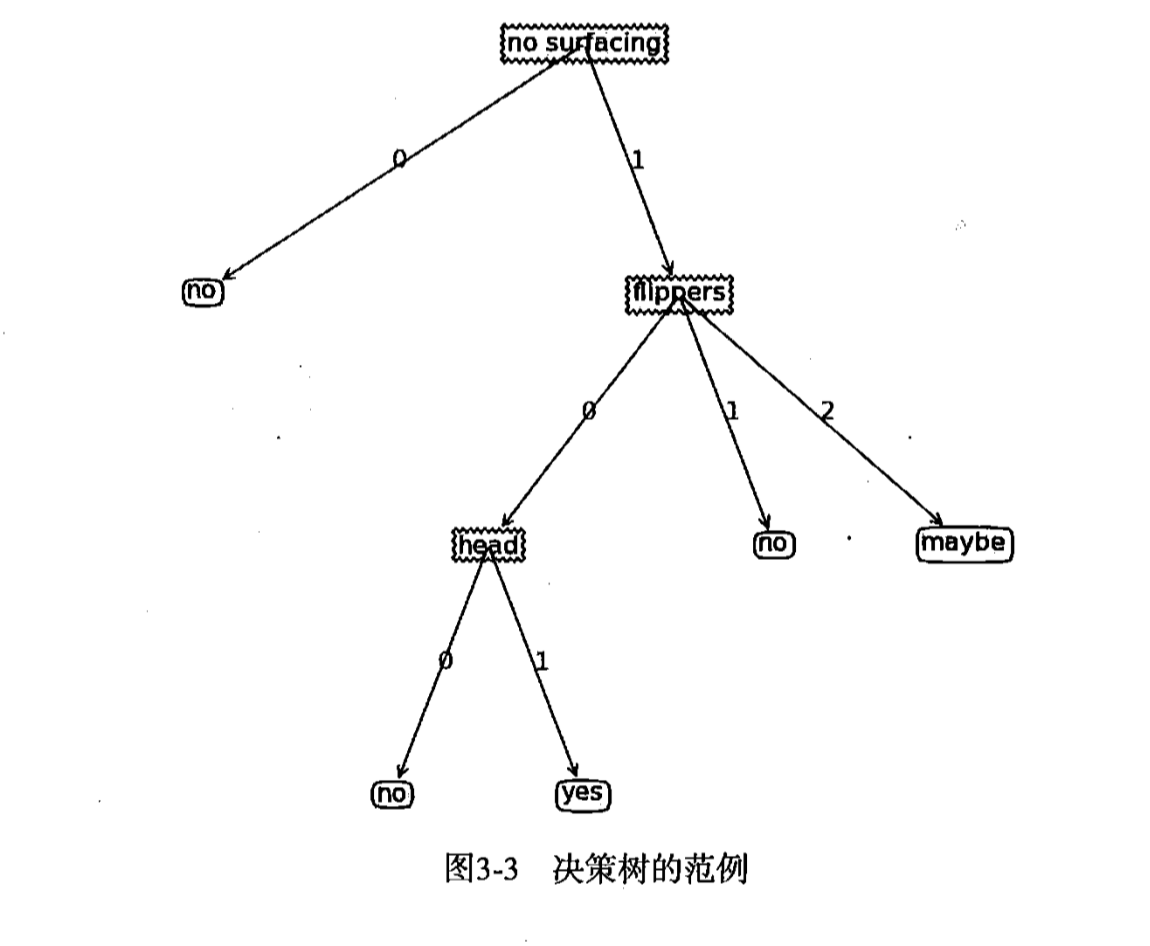matplotlib注解
matplotlib提供了一个注解工具annotion,非常有用,它可以在数据图形上添加文本注释。注解通常用于解释数据的内容。由于数据上面直接存在文本描述非常丑陋,因此工具内嵌支 持带箭头的划线工具,使得我们可以在其他恰当的地方指向数据位置,并在此处添加描述信息, 解 释 数 据 内 容.

decisionNode = dict(boxstyle="sawtooth", fc="0.8")
leafNode = dict(boxstyle="round4", fc="0.8")
arrow_args = dict(arrowstyle="<-")

def plotNode(nodeTxt, centerPt, parentPt, nodeType):
createPlot.ax1.annotate(nodeTxt, xy=parentPt, xycoords='axes fraction',
xytext=centerPt, textcoords='axes fraction',
va="center", ha="center", bbox=nodeType, arrowprops=arrow_args)

def createPlot():
fig = plt.figure(1, facecolor='white')
fig.clf()
createPlot.ax1 = plt.subplot(111, frameon=False) #ticks for demo puropses
plotNode('a decision node', (0.5, 0.1), (0.1, 0.5), decisionNode)
plotNode('a leaf node', (0.8, 0.1), (0.3, 0.8), leafNode)
plt.show()


def getNumLeafs(myTree):
numLeafs = 0
firstStr = myTree.keys()
secondDict = myTree[firstStr]
for key in secondDict.keys():
if type(secondDict[
key]).__name__ == 'dict':  # test to see if the nodes are dictonaires, if not they are leaf nodes
numLeafs += getNumLeafs(secondDict[key])
else:
numLeafs += 1
return numLeafs

def getTreeDepth(myTree):
maxDepth = 0
firstStr = myTree.keys()
secondDict = myTree[firstStr]
for key in secondDict.keys():
if type(secondDict[
key]).__name__ == 'dict':  # test to see if the nodes are dictonaires, if not they are leaf nodes
thisDepth = 1 + getTreeDepth(secondDict[key])
else:
thisDepth = 1
if thisDepth > maxDepth: maxDepth = thisDepth
return maxDepth


def plotTree(myTree, parentPt, nodeTxt):  # if the first key tells you what feat was split on
numLeafs = getNumLeafs(myTree)  # this determines the x width of this tree
depth = getTreeDepth(myTree)
firstStr = myTree.keys()  # the text label for this node should be this
cntrPt = (plotTree.xOff + (1.0 + float(numLeafs)) / 2.0 / plotTree.totalW, plotTree.yOff)
plotMidText(cntrPt, parentPt, nodeTxt)
plotNode(firstStr, cntrPt, parentPt, decisionNode)
secondDict = myTree[firstStr]
plotTree.yOff = plotTree.yOff - 1.0 / plotTree.totalD
for key in secondDict.keys():
if type(secondDict[
key]).__name__ == 'dict':  # test to see if the nodes are dictonaires, if not they are leaf nodes
plotTree(secondDict[key], cntrPt, str(key))  # recursion
else:  # it's a leaf node print the leaf node
plotTree.xOff = plotTree.xOff + 1.0 / plotTree.totalW
plotNode(secondDict[key], (plotTree.xOff, plotTree.yOff), cntrPt, leafNode)
plotMidText((plotTree.xOff, plotTree.yOff), cntrPt, str(key))
plotTree.yOff = plotTree.yOff + 1.0 / plotTree.totalD

# if you do get a dictonary you know it's a tree, and the first element will be another dict

def createPlot(inTree):
fig = plt.figure(1, facecolor='white')
fig.clf()
axprops = dict(xticks=[], yticks=[])
createPlot.ax1 = plt.subplot(111, frameon=False, **axprops)  # no ticks
# createPlot.ax1 = plt.subplot(111, frameon=False) #ticks for demo puropses
plotTree.totalW = float(getNumLeafs(inTree))
plotTree.totalD = float(getTreeDepth(inTree))
plotTree.xOff = -0.5 / plotTree.totalW;
plotTree.yOff = 1.0;
plotTree(inTree, (0.5, 1.0), '')
plt.show()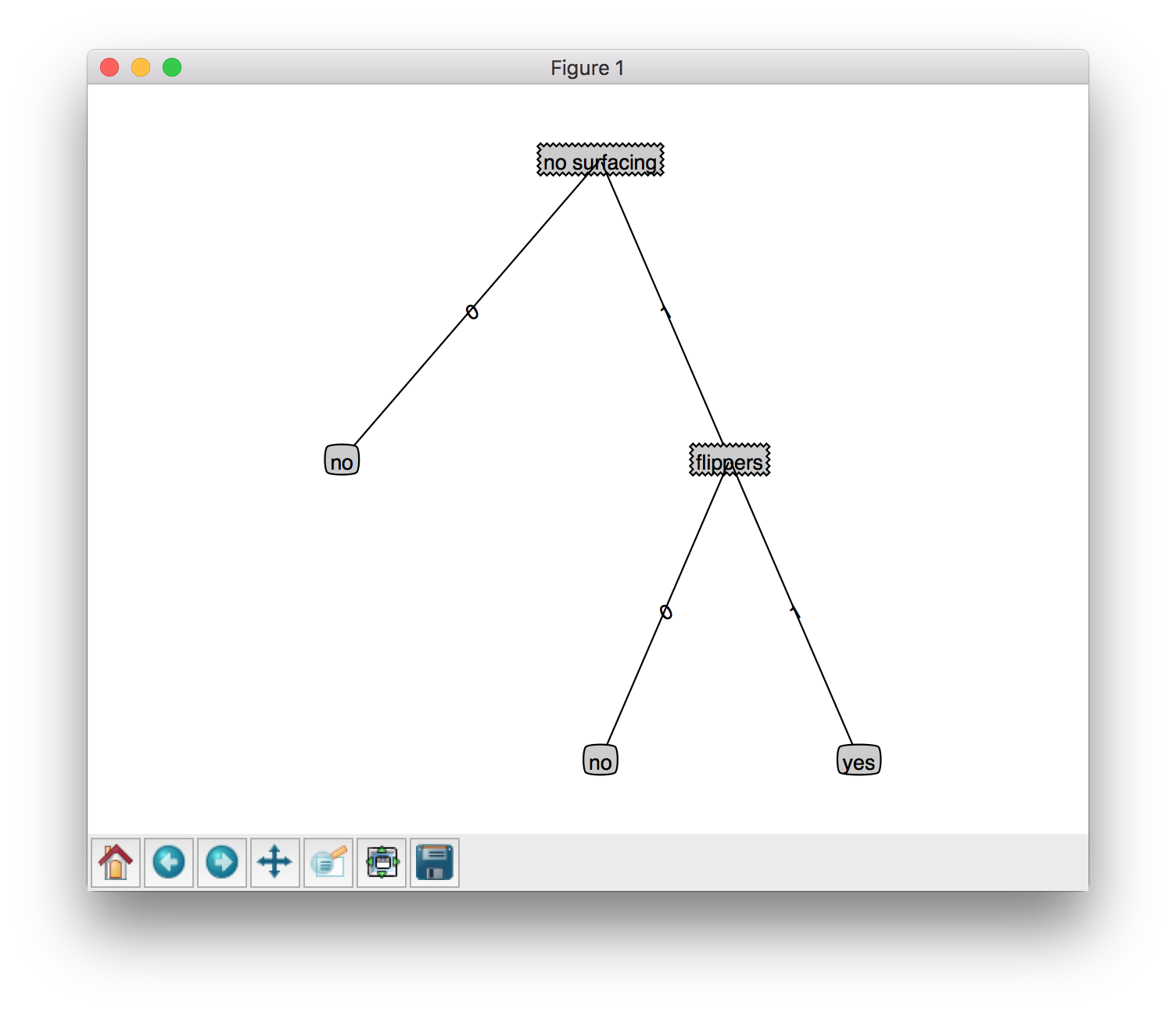#### 测试与存储分类器

def classify(inputTree, featLabels, testVec):
firstStr = inputTree.keys()
secondDict = inputTree[firstStr]
featIndex = featLabels.index(firstStr)
key = testVec[featIndex]
valueOfFeat = secondDict[key]
if isinstance(valueOfFeat, dict):
classLabel = classify(valueOfFeat, featLabels, testVec)
else:
classLabel = valueOfFeat
return classLabel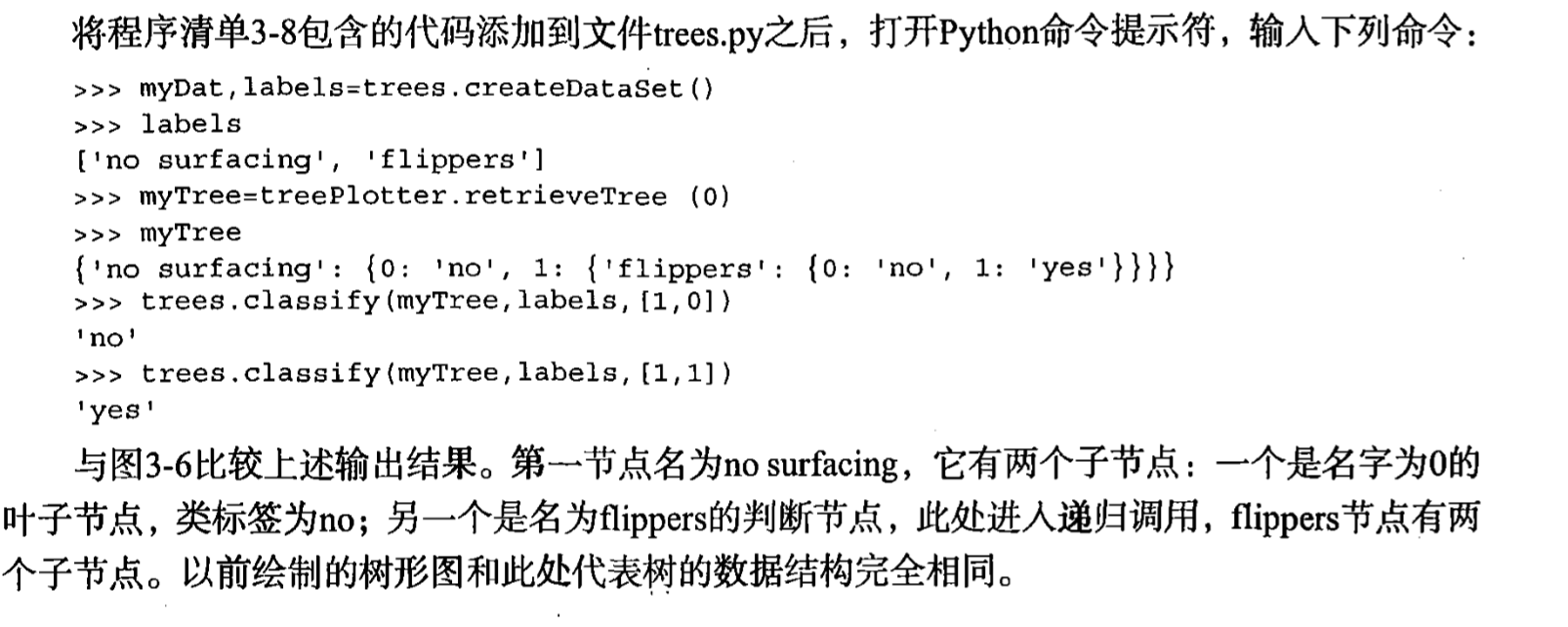def storeTree(inputTree, filename):
import pickle
fw = open(filename, 'w')
pickle.dump(inputTree, fw)
fw.close()

def grabTree(filename):
import pickle
fr = open(filename)


def glassTest():
fr = open('lenses.txt')
lenses = [inst.strip().split('\t') for inst in fr.readlines()]
lensesLabels = ['age','prescript','astigmatic','tearRate']
lensesTree = createTree(lenses,lensesLabels)
treePlot.createPlot(lensesTree)